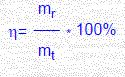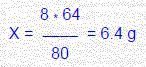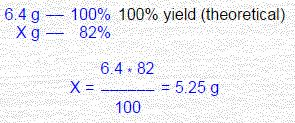# What is The Law of Conservation of Mass?

The mass of the substances entering into a reaction equal the mass of the substances formed as a result of the reaction. (M.V. Lomonosov, 1748, A. Lavoisier 1789)

Atomic-molecular concept explains this law by following manner: as a result of chemical reactions atoms do not appear and do not disappear, but occurs their rearrangement (i.e. chemical conversion-process of bond breakup between atoms with another bond formation, as a result of such conversation the molecules of source substances transform into the molecules of products of reaction). As far as a number of atoms before and after reaction stays unchangeable their general mass also must not change. At term «mass» has understood a value-characterizing amount of matter.

At the beginning of 20 century a formulation of mass conversation law was revising in connection with the appearance of relativity theory (A. Einstein, 1905), according to which the mass of substance depends on its velocities, and consequently characterizes not only matter amount, as well as its motion. An Energy E, obtained by substance, is connected with increasing its mass Dm by the correlation, E = M * C^2, where c is a light velocity. This correlation is not used in chemical reactions, since 1k J of energy is corresponding ~ 10^-11g mass changing and practically cannot be measured.

In nucleus reactions, where E greater in 106 times than in chemical reactions, M should take into account.
Originating from the mass conservation law equations of chemical reactions is possible to form with the following calculations. It is a basis of quantitative chemical analysis.

Arranging of chemical equations

Include three stages:

1. Record formulas of substances: entered in the reaction (on the left) and products of reaction (on the right), having connected them on the sense by signs «+», «®»:2. Selection the coefficients for each substance so that amount of atoms of each element in left and right part of equation will be equally:3. Checking a number of atoms of each element in left and right parts of equation.

Calculations on chemical equations

Calculations on chemical equations (stoichiometric calculations) are based on the mass conservation law of substances. In real chemical processes because of incomplete running chemical reactions and losses a products mass usually less theoretically calculated.
Yield of reaction (n) is a ratio of real product mass (mr) to theoretically possible (mt), expressed in shares of units or percent.
If in conditions of problems a yield of reactions products does not specified, its take in calculations as 100% (quantitative output).example

Calculated the mass of copper formed when reduction 8 g copper oxide by hydrogen. The yield of reaction – 82% from theoretical.

Solution:1. Calculate a theoretical yield of copper on the equation of reaction: 80 g (1 g-mol) CuO when reducing can form 64 g (1 g-mol) Cu. 8 g CuO – X g Cu.2. Define how much grams of copper will be formed at 82% yield of product